PERT Math Practice Test

Try our free PERT Math practice test. These practice question are designed to be similar to those found on the math section of Florida’s Postsecondary Education Readiness Test. Topics covered include equations, exponents, percents, prime numbers, polynomials, order of operations, and algebraic expressions. The PERT Math test includes 30 questions and there is no time limit. Start your test prep right now with our free practice test!

Congratulations - you have completed .

You scored %%SCORE%% out of %%TOTAL%%.

Your performance has been rated as %%RATING%%

 Question 1
If y = 3ab + 2, what is y when a = 1 and b = 2?

 A 16 B 18 C 20 D 22
Question 1 Explanation:
The correct answer is (D). Substitute the given values for a and b into the equation and then evaluate to find y:

y = 3ab + 2b³
y = 3(1)(2) + 2(2³)
y = 6 + 16
y = 22
 Question 2
What is 181.5% of 18?

 A 3.267 B 14.67 C 32.67 D 3267
Question 2 Explanation:
The correct answer is (C). Recall that the % symbol means ‘per hundred.’ So, 181.5% is equivalent to 181.5/100 or 1.815. Converting a percentage to a decimal always entails moving the decimal point two places to the left. Multiply this decimal value with 18 to answer the question:

1.815 × 18 = 32.67
 Question 3
Solve for x:

3(x + 1) = 5(x − 2) + 7

 A −2 B 2 C ½ D 3
Question 3 Explanation:
The correct answer is (D). Begin by distributing the 3 and the 5 through their respective parentheses, then combine like terms on each side of the equal sign:

3(x + 1) = 5(x − 2) + 7
3x + 3 = 5x − 10 + 7
3x + 3 = 5x − 3

Add 3 to both sides to maintain the equality:

3x + 6 = 5x

Subtract 3x from both sides and then divide the resulting equation by 2 to solve for x as follows:

6 = 2x
3 = x
 Question 4
The data in the table below shows the results of Tracy trying to train her dog Snowy.In what percentage of these trials did Snowy obey the command given?

 A 25% B 37.5% C 50% D 62.5%
Question 4 Explanation:
The correct answer is (C). Calculate the percentage of trials that Snowy obeyed by dividing the number of times Snowy obeyed by the total number of commands given. The number of times Snowy obeyed: trial 1, trial 3, trial 6, trial 8, which sums to 4 trials. There are 8 total trials so the percentage is: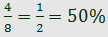Question 5
Which of the following expressions is equivalent to 3x(2 + 5y) ?

 A 3x + 5y B 6x + 5y C 6x + 15y D 6x + 15xy
Question 5 Explanation:
The correct answer is (D). Begin by assessing the form in which the answer choices are written. As no answer choice contains a parenthetical expression, start by distributing the 3x through the parentheses. Multiply 3x by each of the terms inside of the parentheses and combine any like terms to simplify:

3x(2 + 5y)
= 3x(2) + 3x(5y)
= 6x + 15xy
 Question 6
What is the value of the expression given below?

26 − 7(3 + 5) ÷ 4 + 2

 A 14 B 16.67 C 23 D 40
Question 6 Explanation:
The correct answer is (A). We can use PEMDAS to evaluate the expression. (PEMDAS is a technique for remembering the order of operations. It stands for Parentheses, Exponents, Multiplication and Division, and Addition and Subtraction) According to the rules, we must evaluate the expression in the parentheses first:

26 − 7(3 + 5) ÷ 4 + 2
= 26 − 7(8) ÷ 4 + 2

Then we perform multiplication and division in order from left to right as follows:

26 − 7(8) ÷ 4 + 2
= 26 − 56 ÷ 4 + 2
= 26 − 14 + 2

Finally, we perform addition and subtraction in order from left to right as follows:

26 − 14 + 2
= 12 + 2
= 14
 Question 7
What percentage of the squares are shaded in the grid shown below?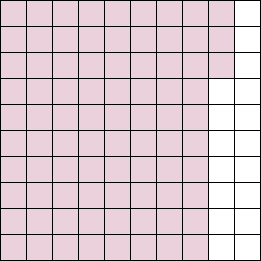A 17% B 93% C 83% D 73%
Question 7 Explanation:
The correct answer is (C). The percentage of squares that are shaded can be expressed as the ratio of the number of shaded squares to the total number of squares all multiplied by 100%, stated algebraically: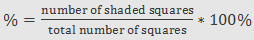And, substituting the values:Question 8
How many of the numbers between 10 and 25 are prime numbers?

 A 4 B 5 C 6 D 7
Question 8 Explanation:
The correct answer is (B). A prime number is a number with no factors other than 1 and itself. Therefore, we can solve this problem by listing the numbers from 10 to 25 and eliminating those which are clearly divisible by some other number.

It is only necessary to list the odd numbers in this range, since the even numbers are all divisible by 2:

11, 13, 15, 17, 19, 21, 23, 25

Eliminate numbers divisible by 3: 15, 21
Eliminate numbers divisible by 5: 25

A quick check will tell us that none of the remaining numbers are divisible by anything other than themselves and 1. That leaves: 11, 13, 17, 19, and 23, for a total of 5 numbers.
 Question 9
In the coordinate plane shown, which point represents (−1, 3)?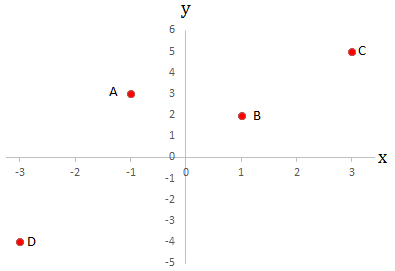A A B B C C D D
Question 9 Explanation:
The correct answer is (A). The coordinate plane has a horizontal x axis and a vertical y axis that intersect at the point (0,0). The x values along the x axis increase to the right and decrease to the left, with negative values to the left of 0. The y values along the y axis increase vertically upwards and decrease vertically downwards, with negative values below 0.

The point (−1, 3) is then 1 unit to the left of (0, 0), and 3 units upwards from (−1, 0). This is represented by point A on the coordinate plane.
 Question 10
Simplify: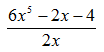A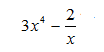B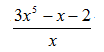C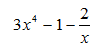D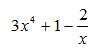Question 10 Explanation:
The correct answer is (C). Completely simplify the expression by dividing every term in the numerator by the term in the denominator: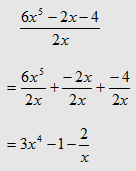Question 11
Simplify:

(7y2 + 3xy − 9) − (2y2 + 3xy − 5)

 A 5y2 − 4 B 9y2 + 6xy − 14 C 5y2 + 4 D 5y2 + 6xy − 14
Question 11 Explanation:
The correct answer is (A). When subtracting polynomials, the first step is to distribute the negative sign through the parentheses. This changes the sign on each term inside the parentheses:

7y2 + 3xy − 9 − 2y2 − 3xy + 5

Arrange like terms next to each other (optional):

7y2 − 2y2 + 3xy − 3xy − 9 + 5

Combine like terms to compute the answer:

5y2 − 4
 Question 12
What is the slope of the line that passes through points (0,5) and (7,0) on the coordinate plane shown below?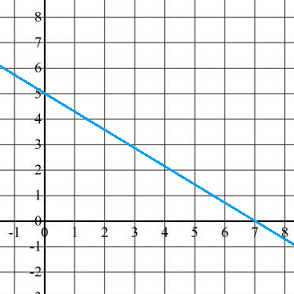ABC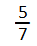D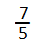Question 12 Explanation:
The correct answer is (B). The slope of a line, which can be remembered as “the rise over the run,” is the change in y values divided by the change in x values across two points on a line. Written algebraically, the slope is defined as: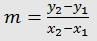Substitute the given points (0,5) and (7,0) into the equation to calculate the slope:Question 13
If Jayla left a \$10.16 tip on a breakfast that cost \$86.89, approximately what percentage was the tip?

 A 12% B 13% C 17% D 25%
Question 13 Explanation:
The correct answer is (A). If you want to know what percent A is of B, you divide A by B, then take that number and move the decimal place two spaces to the right:

10.16 ÷ 86.89 = 0.1169

Move the decimal two spaces to the right to find the percentage:

= 11.69%

This is approximately equal to 12%.
 Question 14
Which of the following expressions is equivalent to (7x + 3y)(8x + 5y)?

 A 7x2 + 23xy + 5y2 B 7x2 + 24xy + 8y2 C 56x2 + 59xy + 15y2 D 56x2 + 35xy + 15y2
Question 14 Explanation:
The correct answer is (C). Use the distributive property to multiply each term in the first parentheses through the second parentheses. When dealing with binomial expressions (those of the form (x ± y)(x ± y)), this method is commonly referred to as the “FOIL method”: multiply the First two terms, then the Outer two terms, then the Inner two terms, and then the Last two terms. Lastly, combine like terms to calculate the answer:

(7x + 3y)(8x + 5y)
56x2 + 35xy + 24xy + 15y2
56x2 + 59xy + 15y2
 Question 15
Which graph represents the solution set of the inequality x +3 > 6?

 A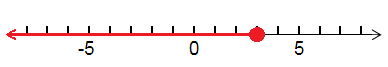B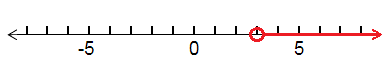C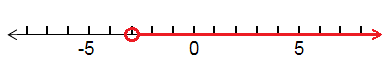DQuestion 15 Explanation:
The correct answer is (B). Begin by noticing that the inequality is a greater than or less than sign, and NOT a greater than or equal to or less than or equal to sign; this indicates that the graph contains an open circle (indicating that the value at the circle is not included as part of the solution set).

Next, isolate the variable by subtracting 3 from both sides: x > 3, which indicates that the solution set includes all x values larger than, but not including x = 3.
 Question 16
Simplify:

(x6)(x5)

 A x11 B 2x30 C x1 D 2x11
Question 16 Explanation:
The correct answer is (A). Recall that the multiplication of terms sharing the same base can be simplified by adding the exponents:

xa * xb = x(a + b).

If this rule is forgotten, you can quickly derive it by considering the simple case of 21 * 22, which can be rewritten as 2 * 2 * 2, or 23. The expression in this case, then, can be simplified as:

(x6) (x5) = x5+6
= x11
 Question 17
This pie chart shows Al’s monthly expenses. If Al spent \$210 on transportation in one month, how much did he spend on food in that month?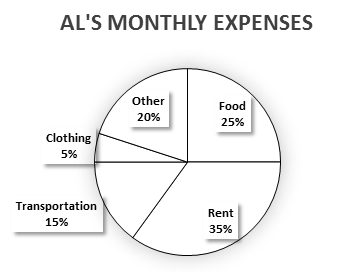A \$126 B \$250 C \$325 D \$350
Question 17 Explanation:
The correct answer is (D). One method for solving this problem is to recognize that the ratio of the amount Al spends on food to the amount he spends on transportation will equal the ratio of the percentage of his income spent on food to the percentage spent on transportation. Expressed mathematically: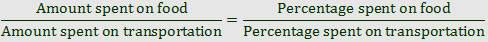Substitute the known values to then solve for the unknown amount spent on food: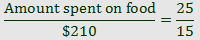Cross multiply and simplify to solve:Question 18
In a coordinate plane, triangle ABC has coordinates: (−2,7), (−3,6), and (4,5). If triangle ABC is reflected over the y-axis, what are the coordinates of the new image?

 A (−2,−7), (−3,−6), (−4,−5) B (−2,−7), (−3,−6), (4,−5) C (2,7), (3,6), (−4,5) D (2,7), (3,6), (4,5)
Question 18 Explanation:
The correct answer is (C). One way to solve this problem is to draw the figure and then count how many units each point is from the y-axis, and to then count the same number of units in the opposite direction to find each point’s reflection. A more efficient method is to recognize that reflecting over the y-axis causes the x-value to switch sign and does not influence the y-value of the point.

Reflecting (−2,7), (−3,6), (4,5) across the y-axis produces the points (2,7), (3,6), (−4,5).
 Question 19
What is the equation of the line that passes through (4, –1) and has a slope of –5?

 A y = –5x + 19 B y = –5x + 21 C y = –5x – 19 D y = –5x – 21
Question 19 Explanation:
The correct answer is (A). The general slope-intercept form of the equation of a line is y = mx + b, where m is the slope and b is the y-intercept.

By substitution of the given point and given slope, we have:

–1 = (–5)(4) + b

So, b = –1 + 20 = 19, and the required equation is y = –5x + 19.
 Question 20
Solve: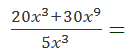A 6 + 6x6 B 4 + 6x6 C 4x3 + 6x9 D 4x3 + 6x3
Question 20 Explanation:
The correct answer is (B). Begin every problem involving polynomials in the numerator or denominator by considering the factoring options. Here, the numerator can be factored as there is a coefficient and variable common to both terms: 10x3. However, before factoring out the full coefficient, notice that dividing out the full 10 would entail multiplying the resulting 2 back through the numerator. Factor out 5x3 and divide by the denominator to immediately arrive at the answer: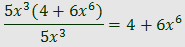Question 21
Oscar purchased a new hat that was on sale for \$5.06. The original price was \$9.20. What percentage discount was the sale price?

 A 4.5% B 41.4% C 45% D 55%
Question 21 Explanation:
The correct answer is (C). The percentage discount is the reduction in price divided by the original price. The difference between original price and sale price is:

\$9.20 − \$5.06 = \$4.14

The percentage discount is this difference divided by the original price:

\$4.14 ÷ \$9.20 = 0.45

Convert the decimal to a percentage by multiplying by 100%:

0.45 × 100% = 45%
 Question 22
Factor: 4y(3x + 2) − 2(3x + 2)

 A (4y − 2)(3x + 2) B (3x − 2)(4y + 2) C (4y + 2)(6x) D (3x + 2)(4y + 2)
Question 22 Explanation:
The correct answer is (A). Any part of an expression that is common to every term in the expression can be factored out. In this case, (3x + 2) is a common term and can be factored out from both parts of the expression:

4y(3x + 2) − 2(3x + 2) = (3x + 2)[4y − 2]

Notice that distributing (3x + 2) through [4y − 2] yields the original expression; this verifies the answer.
 Question 23
If 4x + 3x − 2(x + 5) = −9, then x = ?

 A −1/2 B 0 C 1/5 D 2/3
Question 23 Explanation:
The correct answer is (C). Evaluate the expression by first distributing the −2 through the parentheses and then combining like terms:

4x + 3x − 2x – 10 = −9
7x − 2x − 10 = −9
5x = 1
x = 1/5
 Question 24
Simplify: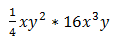A 8x3y B 4x4y3 C 4x3y4 D 8x3y4
Question 24 Explanation:
The correct answer is (B). To simplify this expression, first multiply ¼ by 16. Next, use the rules of exponent multiplication to simplify the expression:

x * x3 = x1 + 3 = x4
y2 * y = y2 + 1 = y3

Combining these values gives:

4x4y3
 Question 25
What are the solutions to the equation:  + 8x + 15 = 0

 A (2, 3) B (3, 5) C (−2, −3) D (−3, −5)
Question 25 Explanation:
The correct answer is (D). The solutions to an equation are those values that when substituted into the equation yield a true statement. As a result, one straightforward method for solving problems like this is to plug each answer choice into the equation to see which values make the equation true.

Alternatively, the values can be calculated by factoring and solving the equation, in this case a quadratic equation.

A quadratic equation is any equation of the form: y = ax² + bx + c, where a, b, and c are known values.

One method for solving a quadratic equation is by factoring the equation and setting each of the factors equal to 0. Not all quadratic equations can be solved by factoring, and in such cases, it is necessary to use the quadratic formula to find the factors.

To simplify the quadratic equation given, two factors that multiply to 15 and add to 8 are necessary. The only valid pair of factors is 3 and 5. The equation can then be rewritten as:

(x + 3)(x + 5) = 0

Recall that if ab = 0, then either a = 0, or b = 0. So, the values of x can be found by setting each parentheses equal to 0 and solving for x:

(x + 3) = 0  or  (x + 5) = 0
x = −3  or  x = −5

Remember that these solutions can be verified by replacing x with the found values and showing that the expression on the left-hand side of the equation is the same as the expression on the right-hand side of the equation.
 Question 26
ABC is a triangle with coordinates (3, 1), (6, 1), and (1, 3). It is translated to points A′(−3, 3), B′( 0, 3), and C′(−5, 5). Find the rule for the translation.

 A 6 units right and 2 units down B 2 units left and 6 units up C 6 units down and 2 units right D 6 units left and 2 units up
Question 26 Explanation:
The correct answer is (D). The rule of translation is the rule, which when applied to the first set of points, yields the second set of points. The difference between the x values of the non-translated and translated points is:

(x2x1) = (−3 − 3) = (0 − 6) = (−5 − 1) = −6

This corresponds to a shift of 6 units to the left.

Likewise, for the y values:

(y2y1) = (3 − 1) = (3 − 1) = (5 − 3) = 2

This corresponds to a vertical shift of 2 units.
 Question 27
What is the equation of a line that passes through points A (1, 2) and B (4, 3)?

 A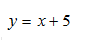B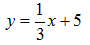C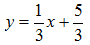D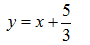Question 27 Explanation:
The correct answer is (C). This problem can be solved by combining two formulas: the formula for the slope of a line and the point-slope form of the equation of a line. Use the two given points to first calculate the slope, then substitute one of the given points and the slope into the point-slope form of a line and simplify. Begin by finding the slope, which is the change in the y coordinates divided by the change in the x coordinates: :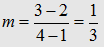Using point A (1, 2), write the equation of the line as follows, where (x1, y1) are the coordinates of a known point: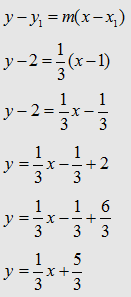Question 28
Which of the following expressions is equivalent to 4y – 7 > 8y – 1?

 A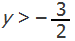B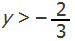C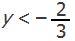D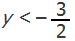Question 28 Explanation:
The correct answer is choice (D). Subtract 4y from each side of the given inequality to find –7 > 4y – 1. Next, add 1 to each side to find –6 > 4y. The last step is to divide both sides by 4, which gives: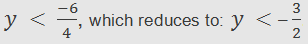Question 29
Which of the following numbers is the greatest?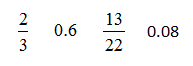A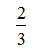B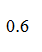CD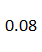Question 29 Explanation:
The correct answer is (A). To determine which value is the greatest, convert the fractions to decimals and compare each value. The fraction 2/3 is equivalent to 2 ÷ 3 or 0.66; the fraction 13/22 evaluates to 0.59.
 Question 30
Given the simultaneous equations x + 2y = –2 and 3x + 4y = 2, what is the value of y?

 A 6 B 0 C –2 D –4
Question 30 Explanation:
The correct answer is (D). Systems of equations can be solved using many techniques. In this case, we will use the method of elimination, which entails multiplying one of the equations by an integer value such that combining both equations eliminates one of the variables (in some cases the equations can be directly combined to eliminate a variable, and the multiplication step is unnecessary).

By multiplying the first equation, x + 2y = –2, by –3, the equations can be added to produce a new equation that is only in terms of y. Multiplying by –3 gives:

–3(x + 2y = –2)
–3x – 6y = 6

And, adding this equation to the second equation to eliminate the x variable:

–3x – 6y + 3x + 4y = 6 + 2
–2y = 8
y = –4
Once you are finished, click the button below. Any items you have not completed will be marked incorrect.
There are 30 questions to complete.
 ← List →